# Upcoming Important Dates

Thursday, December 3 - Parent Virtual Trivia Night
Friday, December 4 - Virtual First Friday
Monday, December 21 - Friday, January 1 - Winter Break

 table div table+table+table+table div table{width:100%;padding:0}table div table+table+table+table div table img{width:96.23%;padding:0;float:none}table div table+table+table+table div table td{width:100%;padding:0 1.88% 18px}/* styles */▪ Giving Tuesday: The 2020-2021 Annual Appeal
▪ Parent Virtual Trivia Night
▪ Virtual First Friday
▪ AP Exam and Payment Information
▪ Winter Break
▪ UChicago Collegiate Scholars Virtual Information Session
▪ Liberal Arts at Drexel: Building 21st Century Skills for the Next Generation of Leaders
▪ Academic Approach SAT Test Prep
▪ College Application and Essay Resources
▪ College Representative Visits
▪ Financial Aid Resources
▪ FON Senior Parent College Night Highlights
▪ Photo Submissions Requested for NCP Stampede!
▪ Order Your 2020-2021 NCP Yearbook
▪ Ways for Parents to Stay in Touch
 ▪ Giving Tuesday: The 2020-2021 Annual Appeal
 ▪ Parent Virtual Trivia Night
 ▪ Virtual First Friday
 ▪ AP Exam and Payment Information
 ▪ Winter Break
 ▪ UChicago Collegiate Scholars Virtual Information Session
 ▪ Liberal Arts at Drexel: Building 21st Century Skills for the Next Generation of Leaders
 ▪ Academic Approach SAT Test Prep
 ▪ College Application and Essay Resources
 ▪ College Representative Visits
 ▪ Financial Aid Resources
 ▪ FON Senior Parent College Night Highlights
 ▪ Photo Submissions Requested for NCP Stampede!
 ▪ Order Your 2020-2021 NCP Yearbook
 ▪ Ways for Parents to Stay in Touch
 table div table+table+table+table+table+table div table{width:100%;padding:0}table div table+table+table+table+table+table div table img{width:96.23%;padding:0;float:none}table div table+table+table+table+table+table div table td{width:100%;padding:0 1.88% 18px}/* styles */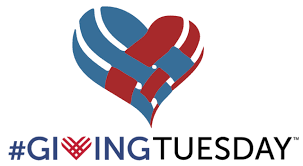# In the Spirit of Giving Tuesday, Donate to the Annual Appeal

## A Global Day of Giving

#GivingTuesday connects diverse groups of individuals, communities, and organizations around the world for one common purpose: to celebrate and encourage giving.

#GivingTuesday is about ordinary people coming together to do extraordinary things. Make a donation to the Annual Appeal and you will help us do extraordinary things for your student and Northside!

## You don't have to wait until Tuesday; donate today!

We still have a long way to go to reach our goal of 100% participation by NCP families and \$500,000!

Employer donations can double or triple the impact of your donation. That means your \$50 donation can result in up to \$150 for Northside! If you aren't sure if your company matches employee contributions, contact your human resources department.

We still have a long way to go. Don't delay! Please donate today.

Keep Northside strong! All donations are appreciated!

 table div table+table+table+table+table+table+table+table+table+table+table div table{width:100%;padding:0}table div table+table+table+table+table+table+table+table+table+table+table div table img{width:96.23%;padding:0;float:none}table div table+table+table+table+table+table+table+table+table+table+table div table td{width:100%;padding:0 1.88% 18px}/* styles */# Parent Virtual Trivia Night

Thursday, December 3
7:30 pm
Zoom Meeting

All are welcome to attend this online event! Grab a snack and join in the fun online. No trivia knowledge needed.

A Zoom link will be shared in a separate e-mail 24 hours before the event.

 table div table+table+table+table+table+table+table+table+table+table+table+table+table div table{width:100%;padding:0}table div table+table+table+table+table+table+table+table+table+table+table+table+table div table img{width:96.23%;padding:0;float:none}table div table+table+table+table+table+table+table+table+table+table+table+table+table div table td{width:100%;padding:0 1.88% 18px}/* styles */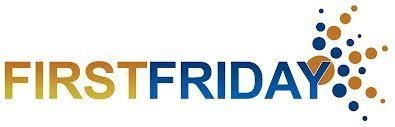# First Friday virtual gathering

Friday, December 4
9:00 am Zoom Meeting

Each month, NCP parents and guardians are invited to get together for a monthly coffee gathering. This month, join fellow NCP parents online. Stay for as long as your schedule allows.

## Join Zoom Meeting

▪ Meeting ID: 848 7451 7349
▪ Passcode: 336446

 table div table+table+table+table+table+table+table+table+table+table+table+table+table+table+table div table{width:100%;padding:0}table div table+table+table+table+table+table+table+table+table+table+table+table+table+table+table div table img{width:96.23%;padding:0;float:none}table div table+table+table+table+table+table+table+table+table+table+table+table+table+table+table div table td{width:100%;padding:0 1.88% 18px}/* styles */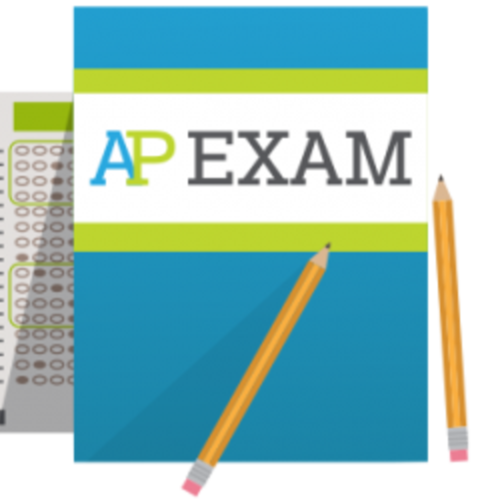# AP Exam and Payment Information

There continues to be a technical issue with the collection of AP Exam fees. In prior communication, AP Exam fee payments would be due by Friday, December 4 at 3:00 pm. Currently, the payment deadline may be adjusted and extended beyond December 4.

An announcement will be made to all students, teachers, and families to ensure that you are able to pay on time. Your continued patience is very much appreciated.

As noted in prior Sunday emails, AP Exams will take place May 3-7 and May 10-14. For reference, the AP exam cost is \$110 per exam. If you completed your family income form and have been approved, the exam cost is \$15 per exam. For students enrolled in AP Seminar and Research, the exam cost is \$143.

 table div table+table+table+table+table+table+table+table+table+table+table+table+table+table+table+table+table div table{width:100%;padding:0}table div table+table+table+table+table+table+table+table+table+table+table+table+table+table+table+table+table div table img{width:96.23%;padding:0;float:none}table div table+table+table+table+table+table+table+table+table+table+table+table+table+table+table+table+table div table td{width:100%;padding:0 1.88% 18px}/* styles */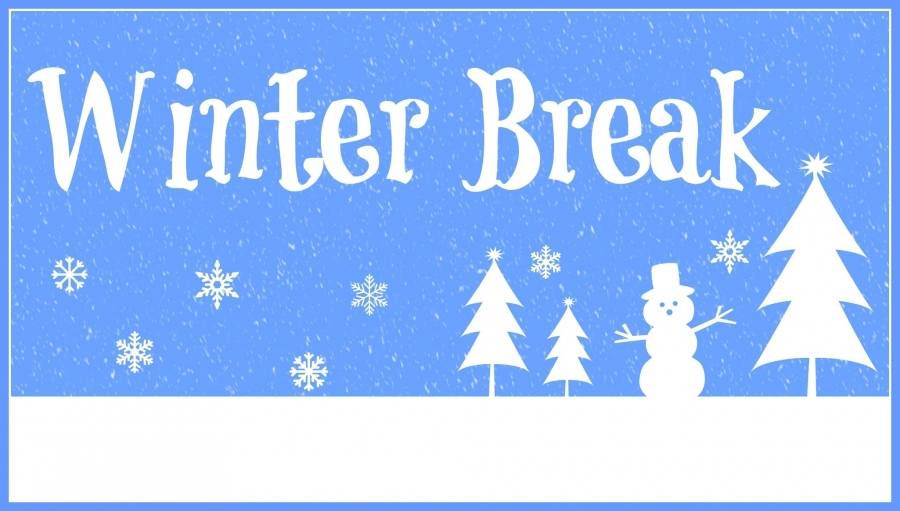# Winter Break

Monday, December 21, 2020 - Friday, January 1, 2021

*Classes resume on Monday, January 4, 2021.

 table div table+table+table+table+table+table+table+table+table+table+table+table+table+table+table+table+table+table+table div table{width:100%;padding:0}table div table+table+table+table+table+table+table+table+table+table+table+table+table+table+table+table+table+table+table div table img{width:96.23%;padding:0;float:none}table div table+table+table+table+table+table+table+table+table+table+table+table+table+table+table+table+table+table+table div table td{width:100%;padding:0 1.88% 18px}/* styles */# Any Questions About NCP Communications Sent Via CNXT?

If you are having problems with SchoolCNXT, please contact the main office from 7:30 am - 3:15 pm at 773-534-3954, or you can email the NCP school clerk, Mary Grasz, at mtgrasz@cpu.edu.

NCP uses SchoolCNXT to communicate important information to parents and the school community. All families should have received a text message and/or email communication about signing up. If you did not receive that communication or need access to your student's account, you can gain access by using this tool on Northside's website.

 table div table+table+table+table+table+table+table+table+table+table+table+table+table+table+table+table+table+table+table+table+table+table div table{width:100%;padding:0}table div table+table+table+table+table+table+table+table+table+table+table+table+table+table+table+table+table+table+table+table+table+table div table img{width:96.23%;padding:0;float:none}table div table+table+table+table+table+table+table+table+table+table+table+table+table+table+table+table+table+table+table+table+table+table div table td{width:100%;padding:0 1.88% 18px}/* styles */# University of Chicago Collegiate Scholars Virtual Information Session

On Wednesday, December 2 at 3:15 pm, the University of Chicago Collegiate Scholars Program will facilitate a virtual information session for 9th-grade NCP students. For more details about the program and registration, review the details below.

Founded in 2003, the University of Chicago Collegiate Scholars Program (CSP) is a three-year program designed to encourage high achieving and underrepresented Chicago Public Schools students to apply to and succeed at highly selective colleges and universities.

CSP is shaped around a core curriculum of humanities, social science, math, and science courses.

During the summer months, scholars are taught by University of Chicago faculty and Ph.D. candidates.

Enrichment activities during the academic year are geared toward college readiness, leadership development, civic engagement, and cultural exploration.

Follow the directions below to register for the Collegiate Scholar virtual information session:

• Login to the Student Naviance homepage https://student.naviance.com/northsideprep

• You will see an active Virtual Visit hyperlink that will provide you with access to the visit. However, for additional security measures, a Google Meeting invitation will be sent to all registered participants in Naviance at least one day before the scheduled virtual visit.

*Please note, all virtual visits will be conducted via CPS Google Meets and no email addresses outside CPS will be permitted into the virtual postsecondary visits. To protect the privacy and safety of students, parents will only be permitted to access virtual visits if their respective student is present and the student will be required to login via their CPS Gmail account. All postsecondary visits will be managed and monitored by an NCP staff member.

If you have any issues registering for the virtual visits, contact Ms. Moore at mcmoore6@cps.edu and she will manually register you for the postsecondary information session.

 table div table+table+table+table+table+table+table+table+table+table+table+table+table+table+table+table+table+table+table+table+table+table+table+table div table{width:100%;padding:0}table div table+table+table+table+table+table+table+table+table+table+table+table+table+table+table+table+table+table+table+table+table+table+table+table div table img{width:96.23%;padding:0;float:none}table div table+table+table+table+table+table+table+table+table+table+table+table+table+table+table+table+table+table+table+table+table+table+table+table div table td{width:100%;padding:0 1.88% 18px}/* styles */# Liberal Arts at Drexel: Building 21st Century Skills for the Next Generation of Leaders

Thursday, December 3
3:00-4:00 pm CST/4:00-5:00 pm EST

Hear from Drexel University Deans - Paula Marantz-Cohen (Pennoni Honors College), Norma Bouchard (College of Arts and Sciences), and Dan Filler (Kline School of Law) - as they showcase the value of the liberal arts at Drexel and the university's commitment to building 21st-century skills for the next generation.

This virtual conversation followed by an alumni panel will include the renowned Deans and Lynne Hickle, Executive Director of Cooperative Education, as they share insights about the importance of the liberal arts within academia, industry, and society. The event will be held via a Zoom webinar.

 table div table+table+table+table+table+table+table+table+table+table+table+table+table+table+table+table+table+table+table+table+table+table+table+table+table+table div table{width:100%;padding:0}table div table+table+table+table+table+table+table+table+table+table+table+table+table+table+table+table+table+table+table+table+table+table+table+table+table+table div table img{width:96.23%;padding:0;float:none}table div table+table+table+table+table+table+table+table+table+table+table+table+table+table+table+table+table+table+table+table+table+table+table+table+table+table div table td{width:100%;padding:0 1.88% 18px}/* styles */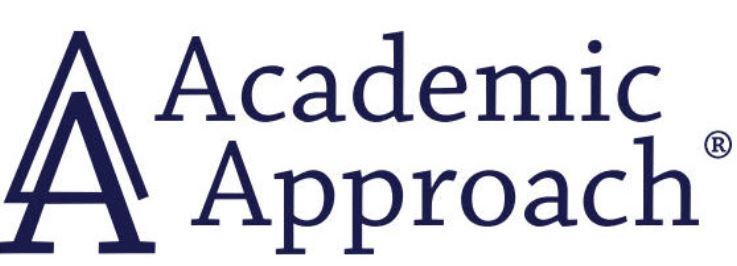# Academic Approach SAT Test Prep

Academic Approach is offering an optional virtual SAT prep course to help NCP juniors and sophomores prepare for the Spring SAT School Day test scheduled for April 2021. It runs from January 20th-March 24, 2021 to help students prepare for the spring SAT in April. Information regarding the schedule, registration details, and course fee is available by following the link below. The deadline to register is December 27, 2020. Click here for more details.

 table div table+table+table+table+table+table+table+table+table+table+table+table+table+table+table+table+table+table+table+table+table+table+table+table+table+table+table+table div table{width:100%;padding:0}table div table+table+table+table+table+table+table+table+table+table+table+table+table+table+table+table+table+table+table+table+table+table+table+table+table+table+table+table div table img{width:96.23%;padding:0;float:none}table div table+table+table+table+table+table+table+table+table+table+table+table+table+table+table+table+table+table+table+table+table+table+table+table+table+table+table+table div table td{width:100%;padding:0 1.88% 18px}/* styles */# College Application and Essay Resources

Hello, Class of 2021 Parents,

Time is moving at light speed and the December college application deadlines are quickly approaching. New York University has you covered with helpful tips and best practices as your students prepare to hit submit on their college applications!

Here are a few articles that will assist you in supporting your students as they tackle their college applications and essays.

If you have any questions or concerns, do not hesitate to contact Ms. Moore at mcmoore6@cps.edu or email your student’s respective school counselor. We are here to support your family with navigating the next steps in the college admissions process.

▪ 3 Tips to Choose the Right Common App Essay Prompt (click here)
▪ What I Wish I Knew Before Applying to College (click here)

 table div table+table+table+table+table+table+table+table+table+table+table+table+table+table+table+table+table+table+table+table+table+table+table+table+table+table+table+table+table+table+table div table{width:100%;padding:0}table div table+table+table+table+table+table+table+table+table+table+table+table+table+table+table+table+table+table+table+table+table+table+table+table+table+table+table+table+table+table+table div table img{width:96.23%;padding:0;float:none}table div table+table+table+table+table+table+table+table+table+table+table+table+table+table+table+table+table+table+table+table+table+table+table+table+table+table+table+table+table+table+table div table td{width:100%;padding:0 1.88% 18px}/* styles */# College Representative Visits

The NCP Counseling Department is offering virtual postsecondary visits for Northside Prep students and parents. We are offering virtual postsecondary visits to ensure all families have the opportunity to explore various postsecondary pathways, engage with the postsecondary representatives, and obtain answers to pertinent questions or concerns. Virtual postsecondary visits are offered after school from 3:15 pm - 4:00 pm, and during Flex Blocks 1-4 on Wednesdays.

To date, college, military, and GAP Year representatives are scheduled to connect with NCP students virtually, and the list is growing every day. Click HERE to view the list of upcoming and past postsecondary visits. Also, check out the messages from the admissions representatives and the additional resources they shared during their visits.

*Please note, all virtual visits will be conducted via CPS Google Meets and no email addresses outside CPS will be permitted into the virtual postsecondary visits. To protect the privacy and safety of students, parents will only be permitted to access virtual visits if their respective student is present and the student will be required to log in via their CPS Gmail account. All postsecondary visits will be managed and monitored by an NCP staff member.

 table div table+table+table+table+table+table+table+table+table+table+table+table+table+table+table+table+table+table+table+table+table+table+table+table+table+table+table+table+table+table+table+table+table div table{width:100%;padding:0}table div table+table+table+table+table+table+table+table+table+table+table+table+table+table+table+table+table+table+table+table+table+table+table+table+table+table+table+table+table+table+table+table+table div table img{width:96.23%;padding:0;float:none}table div table+table+table+table+table+table+table+table+table+table+table+table+table+table+table+table+table+table+table+table+table+table+table+table+table+table+table+table+table+table+table+table+table div table td{width:100%;padding:0 1.88% 18px}/* styles */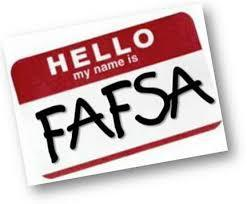# Financial Aid Resources

Video: Q&A with the Director of Financial Aid at Notre Dame (click here): Director of Financial Aid Mary Nucciarone answered questions from students and families during a recent University of Notre Dame Admissions LIVE event. Watch the session on-demand for insights and information about the financial aid process.

How Personal Savings are Considered on the FAFSA (click here): Many families think that saving for college may hurt their chances of getting financial aid. The reality is that the money the average family saves will not significantly affect the amount of aid they receive. There are several reasons why.

Financial Aid Q&A: Take this Chart to Your Next College Visit (click here): This handy chart lists 10 common financial aid questions. Ask them at your college visits and fill in the answers to get a full picture of the financial aid processes at your top-choice schools. We've included our answers too.

Presentations

Net Price Calculators

## Update: Recursos de presentación en Español

▪ Solicitud Alternativa para aplicar para la Subvención MAP de Illinois 2020-2021 (click here)
 ▪ Solicitud Alternativa para aplicar para la Subvención MAP de Illinois 2020-2021 (click here)
 table div table+table+table+table+table+table+table+table+table+table+table+table+table+table+table+table+table+table+table+table+table+table+table+table+table+table+table+table+table+table+table+table+table+table+table+table div table{width:100%;padding:0}table div table+table+table+table+table+table+table+table+table+table+table+table+table+table+table+table+table+table+table+table+table+table+table+table+table+table+table+table+table+table+table+table+table+table+table+table div table img{width:96.23%;padding:0;float:none}table div table+table+table+table+table+table+table+table+table+table+table+table+table+table+table+table+table+table+table+table+table+table+table+table+table+table+table+table+table+table+table+table+table+table+table+table div table td{width:100%;padding:0 1.88% 18px}/* styles */# CPS Scholarship Notifications and College Events Around the City

For more details about upcoming events and to access the most recent updates, (click HERE) to view the College Calendar on the Northside Prep Counseling Website. Also, for additional scholarship opportunities visit the Northside College Prep Counseling page (click HERE).

Tuesday, December 1
App Closes: Act Six Leadership Scholarship

Sunday, December 6
App Closes: Hispanic Heritage Foundation’s Youth Awards

 table div table+table+table+table+table+table+table+table+table+table+table+table+table+table+table+table+table+table+table+table+table+table+table+table+table+table+table+table+table+table+table+table+table+table+table+table+table+table div table{width:100%;padding:0}table div table+table+table+table+table+table+table+table+table+table+table+table+table+table+table+table+table+table+table+table+table+table+table+table+table+table+table+table+table+table+table+table+table+table+table+table+table+table div table img{width:96.23%;padding:0;float:none}table div table+table+table+table+table+table+table+table+table+table+table+table+table+table+table+table+table+table+table+table+table+table+table+table+table+table+table+table+table+table+table+table+table+table+table+table+table+table div table td{width:100%;padding:0 1.88% 18px}/* styles */# FON Senior Parent College Night Highlights

We have written up the highlights of FON’s Senior Parent College Night for all those who were not able to attend. Panelists discussed the college search and application process; financial aid; what virtual graduation was like; college orientation and finding a roommate; the decision of whether or not to attend college courses remotely; and other aspects of attending college during a pandemic. You can find the write-up HERE.

 table div table+table+table+table+table+table+table+table+table+table+table+table+table+table+table+table+table+table+table+table+table+table+table+table+table+table+table+table+table+table+table+table+table+table+table+table+table+table+table+table div table{width:100%;padding:0}table div table+table+table+table+table+table+table+table+table+table+table+table+table+table+table+table+table+table+table+table+table+table+table+table+table+table+table+table+table+table+table+table+table+table+table+table+table+table+table+table div table img{width:96.23%;padding:0;float:none}table div table+table+table+table+table+table+table+table+table+table+table+table+table+table+table+table+table+table+table+table+table+table+table+table+table+table+table+table+table+table+table+table+table+table+table+table+table+table+table+table div table td{width:100%;padding:0 1.88% 18px}/* styles */# Photo Submissions Requested for NCP Stampede!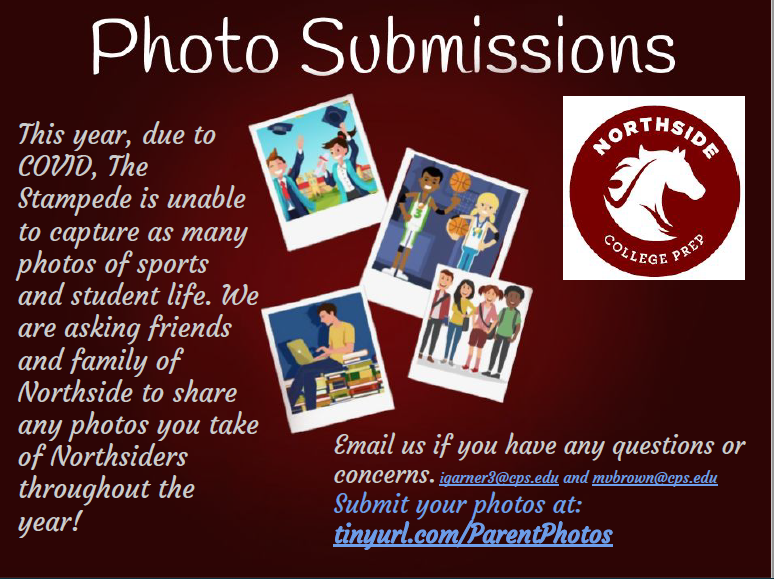table div table+table+table+table+table+table+table+table+table+table+table+table+table+table+table+table+table+table+table+table+table+table+table+table+table+table+table+table+table+table+table+table+table+table+table+table+table+table+table+table+table+table+table div table{width:100%;padding:0}table div table+table+table+table+table+table+table+table+table+table+table+table+table+table+table+table+table+table+table+table+table+table+table+table+table+table+table+table+table+table+table+table+table+table+table+table+table+table+table+table+table+table+table div table img{width:96.23%;padding:0;float:none}table div table+table+table+table+table+table+table+table+table+table+table+table+table+table+table+table+table+table+table+table+table+table+table+table+table+table+table+table+table+table+table+table+table+table+table+table+table+table+table+table+table+table+table div table td{width:100%;padding:0 1.88% 18px}/* styles */# Order a Yearbook Today!

Copies are \$45 until March 1 and \$55 afterwards.

 table div table+table+table+table+table+table+table+table+table+table+table+table+table+table+table+table+table+table+table+table+table+table+table+table+table+table+table+table+table+table+table+table+table+table+table+table+table+table+table+table+table+table+table+table+table+table div table{width:100%;padding:0}table div table+table+table+table+table+table+table+table+table+table+table+table+table+table+table+table+table+table+table+table+table+table+table+table+table+table+table+table+table+table+table+table+table+table+table+table+table+table+table+table+table+table+table+table+table+table div table img{width:96.23%;padding:0;float:none}table div table+table+table+table+table+table+table+table+table+table+table+table+table+table+table+table+table+table+table+table+table+table+table+table+table+table+table+table+table+table+table+table+table+table+table+table+table+table+table+table+table+table+table+table+table+table div table td{width:100%;padding:0 1.88% 18px}/* styles */# Do you have news or team results to share with the Northside parent community?

 table div table+table+table+table+table+table+table+table+table+table+table+table+table+table+table+table+table+table+table+table+table+table+table+table+table+table+table+table+table+table+table+table+table+table+table+table+table+table+table+table+table+table+table+table+table+table+table+table+table div table{width:100%;padding:0}table div table+table+table+table+table+table+table+table+table+table+table+table+table+table+table+table+table+table+table+table+table+table+table+table+table+table+table+table+table+table+table+table+table+table+table+table+table+table+table+table+table+table+table+table+table+table+table+table+table div table img{width:96.23%;padding:0;float:none}table div table+table+table+table+table+table+table+table+table+table+table+table+table+table+table+table+table+table+table+table+table+table+table+table+table+table+table+table+table+table+table+table+table+table+table+table+table+table+table+table+table+table+table+table+table+table+table+table+table div table td{width:100%;padding:0 1.88% 18px}/* styles */# Support Northside - Shop AmazonSmile

AmazonSmile is a simple and automatic way to support Northside every time you shop.

Please note that our AmazonSmile name is Friends of "North" "Side" (North Side - two words at AmazonSmile).

 table div table+table+table+table+table+table+table+table+table+table+table+table+table+table+table+table+table+table+table+table+table+table+table+table+table+table+table+table+table+table+table+table+table+table+table+table+table+table+table+table+table+table+table+table+table+table+table+table+table+table+table div table{width:100%;padding:0}table div table+table+table+table+table+table+table+table+table+table+table+table+table+table+table+table+table+table+table+table+table+table+table+table+table+table+table+table+table+table+table+table+table+table+table+table+table+table+table+table+table+table+table+table+table+table+table+table+table+table+table div table img{width:96.23%;padding:0;float:none}table div table+table+table+table+table+table+table+table+table+table+table+table+table+table+table+table+table+table+table+table+table+table+table+table+table+table+table+table+table+table+table+table+table+table+table+table+table+table+table+table+table+table+table+table+table+table+table+table+table+table+table div table td{width:100%;padding:0 1.88% 18px}/* styles */# Follow Us on Social Media

 table div table+table+table+table+table+table+table+table+table+table+table+table+table+table+table+table+table+table+table+table+table+table+table+table+table+table+table+table+table+table+table+table+table+table+table+table+table+table+table+table+table+table+table+table+table+table+table+table+table+table+table+table+table div table{width:100%;padding:0}table div table+table+table+table+table+table+table+table+table+table+table+table+table+table+table+table+table+table+table+table+table+table+table+table+table+table+table+table+table+table+table+table+table+table+table+table+table+table+table+table+table+table+table+table+table+table+table+table+table+table+table+table+table div table img{width:96.23%;padding:0;float:none}table div table+table+table+table+table+table+table+table+table+table+table+table+table+table+table+table+table+table+table+table+table+table+table+table+table+table+table+table+table+table+table+table+table+table+table+table+table+table+table+table+table+table+table+table+table+table+table+table+table+table+table+table+table div table td{width:100%;padding:0 1.88% 18px}/* styles */table div table+table+table+table+table+table+table+table+table+table+table+table+table+table+table+table+table+table+table+table+table+table+table+table+table+table+table+table+table+table+table+table+table+table+table+table+table+table+table+table+table+table+table+table+table+table+table+table+table+table+table+table+table+table+table div table{width:100%;padding:0}table div table+table+table+table+table+table+table+table+table+table+table+table+table+table+table+table+table+table+table+table+table+table+table+table+table+table+table+table+table+table+table+table+table+table+table+table+table+table+table+table+table+table+table+table+table+table+table+table+table+table+table+table+table+table+table div table img{width:96.23%;padding:0;float:none}table div table+table+table+table+table+table+table+table+table+table+table+table+table+table+table+table+table+table+table+table+table+table+table+table+table+table+table+table+table+table+table+table+table+table+table+table+table+table+table+table+table+table+table+table+table+table+table+table+table+table+table+table+table+table+table div table td{width:100%;padding:0 1.88% 18px}/* styles */table div table+table+table+table+table+table+table+table+table+table+table+table+table+table+table+table+table+table+table+table+table+table+table+table+table+table+table+table+table+table+table+table+table+table+table+table+table+table+table+table+table+table+table+table+table+table+table+table+table+table+table+table+table+table+table+table+table+table div table{width:100%;padding:0}table div table+table+table+table+table+table+table+table+table+table+table+table+table+table+table+table+table+table+table+table+table+table+table+table+table+table+table+table+table+table+table+table+table+table+table+table+table+table+table+table+table+table+table+table+table+table+table+table+table+table+table+table+table+table+table+table+table+table div table img{width:96.23%;padding:0;float:none}table div table+table+table+table+table+table+table+table+table+table+table+table+table+table+table+table+table+table+table+table+table+table+table+table+table+table+table+table+table+table+table+table+table+table+table+table+table+table+table+table+table+table+table+table+table+table+table+table+table+table+table+table+table+table+table+table+table+table div table td{width:100%;padding:0 1.88% 18px}/* styles */